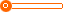# 南非钻石红原瓶进口红酒原装进口红酒原瓶进口葡萄酒_南非红酒南非葡萄酒_神石赤霞珠干红

10000 瓶

10000 瓶

(发货期限：自买家付款之日起 天内发货)

• VIP指数:[第1年]
• 认证信息:
• 所在地区：天津
•• 详细说明
• 规格参数
• 联系方式
r r r r r r r r r r r r 原料与配料:葡萄汁，微量二氧化硫r r r 净重（规格）:750（ml）r r r r r 有无中文标签:有r r r 储藏方法:避光存放。5℃-25℃r r r r r 酒精含量:14%（％）r r r 是否进口:是r r r r r 商品条形码:6009631321205r r r 原产地:南非r r r r r 特产:是r r r 年份:2012r r r r r 包装方式:瓶装r r r 葡萄酒类型:干红葡萄酒r r r r r 葡萄品种:赤霞珠r r r 含糖量:75（g）r r r r r 生产厂家:南非露姿维尔酒庄r r r 产品类别:葡萄酒r r r r r 葡萄汁含量:100（％）r r r 保质期:15（年）r r r r r 原料与配料:葡萄汁，微量二氧化硫r r r 净重（规格）:750（ml）r r r r r 有无中文标签:有r r r 储藏方法:避光存放。5℃-25℃r r r r r 酒精含量:14%（％）r r r 是否进口:是r r r r r 商品条形码:6009631321205r r r 原产地:南非r r r r r 特产:是r r r 年份:2011r r r r r 包装方式:瓶装r r r 葡萄酒类型:干红葡萄酒r r r r r 葡萄品种:赤霞珠r r r 含糖量:75（g）r r r r r 生产厂家:南非露姿维尔酒庄r r r 产品类别:葡萄酒r r r r r 葡萄汁含量:100（％）r r r 保质期:15（年）r r r r r r
r
r
r
r
r
r
r r r r 品名：神石-赤霞珠Bats rock-Cabernet sauvignonr r r 葡萄品种：100％赤霞珠r r r 采摘年份：2011年r r r 原汁含量：100%新鲜葡萄汁r r r 酒精度：14%r r r 产品类型：干红葡萄酒r r

r 产    区：南非西开普-奥勒芬兹r

r

r 产品特点： r

r r       神石是以位于南非西海岸的岩石山脉的名字命名的。这块裸露在地面的岩石当时被早期探险家作为休息的停车点，非常受喜欢并一直受到保护。神石和探险家们都已经不在了，但是他们的开拓精神留在了易饮用、充满果香和容易被人们接受的神石葡萄酒里。神石赤霞珠具有成熟樱桃和柔和白胡椒的香气，还有令人赞美的木梅果香以及烤李子和黑莓香味加之柔和多汁的单宁酸和饱满的酒体，以及令人回味无穷的巧克力，使之成为佳酿。可以搭配番茄等基本菜肴，辛辣食物和红肉。保存在适宜的室内温度。r r r r r r r r r"};

查看更多同类招商加盟产品
上一组 下一组

## 您可以通过以下类目找到类似信息：

免责声明：以上所展示的信息由会员自行提供，内容的真实性、准确性和合法性由发布会员负责。中国酒客网对此不承担任何责任。

友情提醒：为规避购买风险，建议您在购买相关产品前务必确认供应商资质及产品质量！